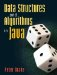This chapter covers some advanced techniques for using linear structures. Section 12.1 introduces bit vectors, an extension of the idea of direct addressing from Section 11.4. Bit vectors are used in an application to help us choose a game to play. Section 12.2 discusses techniques for representing sparse arrays, where almost all of the elements have the same value. Section 12.3 introduces the idea of representing a multidimensional array using a single one-dimensional array. That section concludes with a new implementation of Tic Tac Toe using the ideas from this chapter. Section 12.4 covers some advanced algorithms for searching and sorting.

### Bit VectorsData Structures and Algorithms in Java
ISBN: 0131469142
EAN: 2147483647
Year: 2004
Pages: 216
Authors: Peter Drake